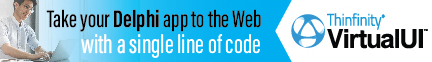DelphiBasicsSqrtFunction Gives the square root of a number System unit
 function Sqrt ( Number :  Extended ) : Extended;
Description
The Sqrt function returns the square root of a Number.

The number must be a floating point type.

Special values are treated as follows:
 Infinity : Infinity -0 : -0 NaN (Not a Number) : NaN
Notes
Warning : the square root of a negative number is an imaginary number. In Delphi, use the Math routines to handle these.

Sqrt should raise an EInvalidOp exception when the Number is negative. In practice, the author's PC crashed (running Windows ME) when attempted.
Related commands
 Sqr Gives the square of a number Sum Return the sum of an array of floating point valuesDownload this web site as a Windows program.